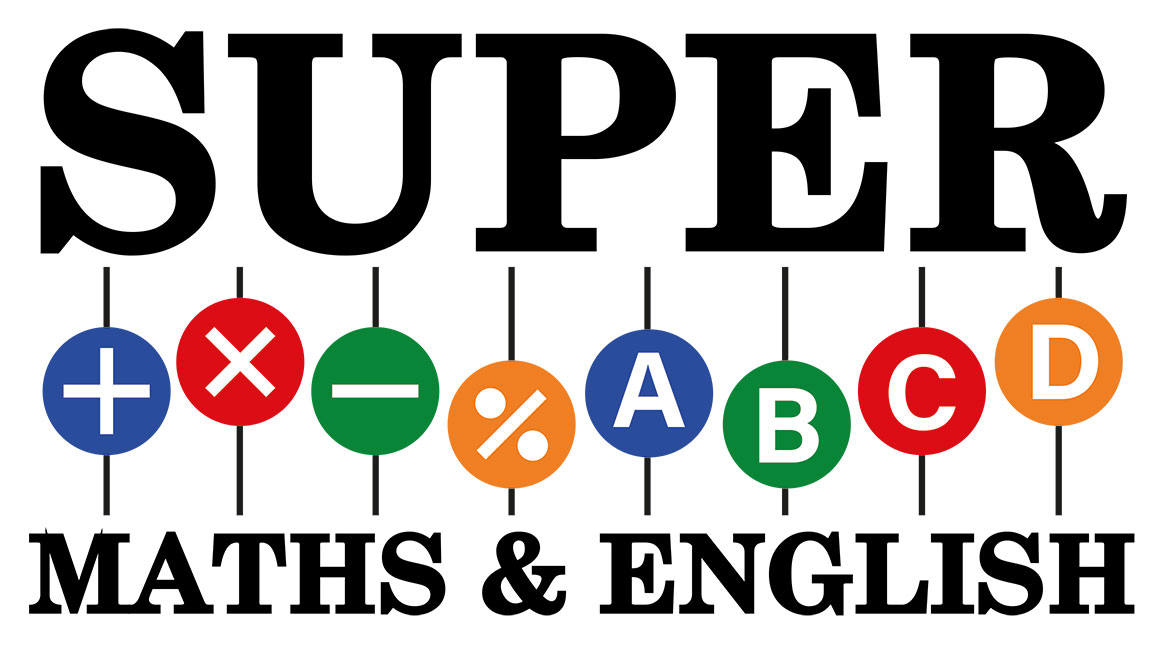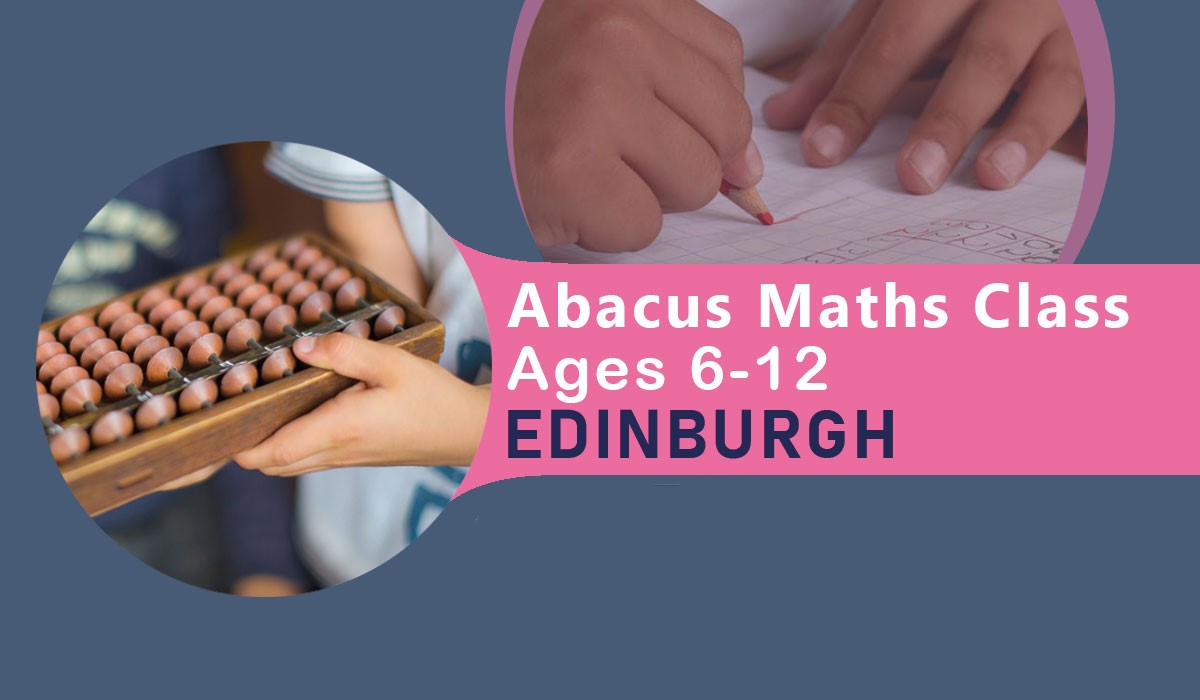Supermaths### Children’s Abacus Maths Class Edinburgh – Every Thursday – 4.30 pm to 6.30 pm

ABACUS Maths class takes place from 4.30 pm to 6.30 pm @Gorgie Dalry Stenhouse Church of Scotland, every Thursday.

## Here is the full address

Gorgie Dalry Stenhouse Church of Scotland

Gorgie Buildings

190-192 Gorgie Rd, Edinburgh, EH11 2NX

0131 337 7936

FEE: One-time registration fee of £35 and £70 per month. Fees is payable in advance.

## What’s the benefit of enrolling in a class?

An Abacus Maths tutor in Edinburgh is a big benefit to your child’s development. If you’ve checked out what Abacus is you know that Maths tutors in Edinburgh are experienced at their job. To learn Abacus in Edinburgh, getting a tutor is essential. We suggest choosing an Abacus Maths tutor in Edinburgh over traditional Maths tutoring. Abacus provides an enriching experience for your child. Abacus Maths tutors in Edinburgh are equipped with all the knowledge and methods required to facilitate and guide your kid.

An Abacus Maths teacher in Edinburgh provides your kid with a collaborative experience. Our teachers are committed to solving all relevant queries and doubts in your kids’ minds. Taking an  Maths tuition in Edinburgh also enhances your child’s time management skills and keeps them in a routine! If you’re going for tuition in Edinburgh for Maths, enrol your child in Abacus Classes in Edinburgh.

All teaching is done in our Glasgow and Edinburgh base- Childrens’ Abacus Maths Classes, Glasgow and Edinburgh.

Here at Super Maths Academy, we offer a child-friendly introduction to the use of the Abacus, both the tool and ultimately the mental theory concepts. We begin with finger theory, move on to an Abacus tool before moving on to mental calculations. Each of our Abacus levels lasts at least 12 weeks. This can differ depending on how well the pupil is coping with the current level.

Maths tutoring in Edinburgh is oriented to give your child support to overcome each level, one step at a time. An abacus Maths teacher in Edinburgh knows the intricacies of each level and prepares your child appropriately for these tests. We provide the best Abacus Maths tutoring in Edinburgh and recommend our Edinburgh Maths classes to you

There are two tracks of the course

1. Junior Track – Junior Track has 11 Levels.
2. Senior Track- Senior Track has 8 Levels.

# Senior Track has 8 Levels and they are detailed as follows:

### Level One

Abacus & finger theory

• 1 to 99 numbers

• +5 combinations

• -5 combinations

• +10 combinations

Mental theory

• No combinations addition and subtraction.

### Level Two

Abacus & Finger theory

• -10 combinations

• double combinations

• double combinations

Abacus

• Multiplication 2 digits x 1 digit

• Times tables 1 to 9

Mental theory

• 1 & 2 digits

• +5 combinations

• -5 combination

• +10 combinations

### Level Three

Abacus

• 2 & 3 digits all combinations

• decimals

• 3 digits double combination

• +10 Combination

• Multiplication 2 digits x 1digit, 3digits x 1 digit and 4 digit x1 digit.

Mental theory

• 2 digits all combinations

• Multiplication 2 digits x 1 digit.

### Level Four

Abacus

• 2,3 & 4 digits all combinations

• decimals 4 digits all combinations

• Multiplication 3 & 4 digits x 1 digit, 2 digits x 2 digits

• Division 2,3 and 4 digits ÷ 1 digit

Mental theory

• 2 & 3 digits all combinations

• decimals 3 digits all combinations

• Multiplication 2,3 and 4 digits x 1 digit,

• Division 2 digits ÷1 digit

### Level Five

Abacus

• 3 & 4 digits all combinations

• Multiplication 2x2digits, 3×2 digits

• Division 3÷1 digits, 4÷1 digit, 3÷2 digits and 4÷2 digits

Mental theory

• 2&3 digits all combinations

• decimal 4 digits all combinations

• Multiplication 3 x 1 digit,4×1 digit 2 x 2 digits

• Division 2÷1 digit, 3÷1 digit, 4÷1 digit

### Level Six

Abacus

• 4 and 5 digits all combinations

• Multiplication 4 x 2 digits, 4×3 digits and 3 x 3 digits

• Division 3÷2 digits, 4÷2 digits and 5÷2 digits

Mental theory

• 2&3 digits all combinations

• decimal 4 digits all combinations

• Multiplication 2x2digits, 3×2 digits and 4×2 digits

• Division 4÷1 digit, 3÷2 digits 4÷2 digits

• Fractions conversion, simple addition and subtraction

### Level Seven

Abacus

• 4 and 5 digits all combinations

• decimal 5 digits all combinations

• Multiplication 3×3 digits, 4×2 digits and 4×3 digits

• Division 4÷2 digits, 5÷2 digits and 5÷3 digits

Mental theory – Addition & subtraction

• 2×3 digits all combinations

• decimal 5 digits all combinations

• Multiplication 3 x 2 digits, 2 x 3 digits, 3×3 digits and 4×3 digits

• Division 4÷2 digits, 5÷2 digits and 5÷3 digits

• Fractions combined operations

• Percentages

### Level Eight

Abacus

• 4 and 5 digits all combinations

• Division 6÷3digits, 5÷3 digits

• Square roots 3 and 4 digits combined operations

Mental theory

• 3 and 4 digits all combinations

• mixed calculations (÷, -, x, +) with bodmas rule

• decimal 5 digits all combinations

• Multiplication 3×2 digits, 3×3 digits

# Junior Track has 11 Levels and they are detailed as follows:

### Level One

Abacus & finger theory

• 1 to 99 numbers

• +5 combinations, -5 combinations (Test week 1 and Test Week 2)

• 2 Digits numbers

### Level Two

Abacus & finger theory

• 1 to 99 numbers

• +10 combinations  (Test week 3 , Test Week 4 and Test Week 5)

• 2 Digits numbers

### Level Three

Abacus & finger theory

• 1 to 99 numbers

• -10 combinations  (Test week 6 , Test Week 7 and Test Week 8)

• 2 Digits numbers

### Level Four

Abacus & finger theory

• 1 to 99 numbers

• +5 combinations

• -5 combinations

• +10 combinations

Mental theory

• No combinations addition and subtraction.

### Level Five

Abacus & Finger theory

• -10 combinations

• double combinations

Abacus

• Multiplication 2 digits x 1 digit

• Times tables 1 to 9

Mental theory

• 1 & 2 digits

• +5 combinations

• -5 combination

• +10 combinations

### Level Six

Abacus

• 2 & 3 digits all combinations

• decimals

• 3 digits double combination

• +10 Combination

• Multiplication 2 digits x 1digit, 3digits x 1 digit and 4 digit x1 digit.

Mental theory

• 2 digits all combinations

• Multiplication 2 digits x 1 digit.

### Level Seven

Abacus

• 2,3 & 4 digits all combinations

• decimals 4 digits all combinations

• Multiplication 3 & 4 digits x 1 digit, 2 digits x 2 digits

• Division 2,3 and 4 digits ÷ 1 digit

Mental theory

• 2 & 3 digits all combinations

• decimals 3 digits all combinations

• Multiplication 2,3 and 4 digits x 1 digit,

• Division 2 digits ÷1 digit

### Level Eight

Abacus

• 3 & 4 digits all combinations

• Multiplication 2x2digits, 3×2 digits

• \

• Division 3÷1 digits, 4÷1 digit, 3÷2 digits and 4÷2 digits

Mental theory

• 2&3 digits all combinations

• decimal 4 digits all combinations

• Multiplication 3 x 1 digit,4×1 digit 2 x 2 digits

• Division 2÷1 digit, 3÷1 digit, 4÷1 digit

### Level Nine

Abacus

• 4 and 5 digits all combinations

• Multiplication 4 x 2 digits, 4×3 digits and 3 x 3 digits

• Division 3÷2 digits, 4÷2 digits and 5÷2 digits

Mental theory

• 2&3 digits all combinations

• decimal 4 digits all combinations

• Multiplication 2x2digits, 3×2 digits and 4×2 digits

• Division 4÷1 digit, 3÷2 digits 4÷2 digits

• Fractions conversion, simple addition and subtraction

### Level Ten

Abacus

• 4 and 5 digits all combinations

• decimal 5 digits all combinations

• Multiplication 3×3 digits, 4×2 digits and 4×3 digits

• Division 4÷2 digits, 5÷2 digits and 5÷3 digits

Mental theory – Addition & subtraction

• 2×3 digits all combinations

• decimal 5 digits all combinations

• decimal 5 digits all combinations

• Multiplication 3 x 2 digits, 2 x 3 digits, 3×3 digits and 4×3 digits

• Division 4÷2 digits, 5÷2 digits and 5÷3 digits

• Fractions combined operations

• Percentages

### Level Eleven

Abacus

• 4 and 5 digits all combinations

• Division 6÷3digits, 5÷3 digits

• Square roots 3 and 4 digits combined operations

Mental theory

• 3 and 4 digits all combinations

• mixed calculations (÷, -, x, +) with bodmas rule

• decimal 5 digits all combinations

• Multiplication 3×2 digits, 3×3 digits

As you can see we have a lot on offer for children to learn, and this will allow them to master their arithmetic skills. You can see videos of our students who are learning the Abacus Maths technique on our YouTube channel, this will allow you to see what is possible within a short span of time. Our Maths tuitions in Edinburgh for Abacus Maths are well suited for all ages. The teachers we have in Edinburgh for Maths tuitions are experienced attending to the specific needs of each student!